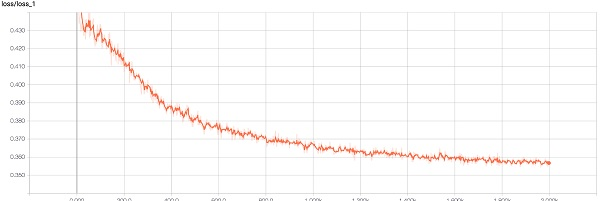Sale!

# Denoising using autoencoders in TensorFlow

19

## Description

Autoencoders can also be used for image denoising. Denoising is the process of removing noise from the image. A denoising encoder can be trained in an unsupervised manner. The noise can be introduced in a normal image and the autoencoder is trained against the original images. Later, the full autoencoder can be used to produce noise-free images. In this section, we will see step-by-step instructions to denoise MNIST images. Import the required libraries and define the placeholders as shown:

```x_input = tf.placeholder(tf.float32, shape=[None, input_size])
y_input = tf.placeholder(tf.float32, shape=[None, input_size])```

Both x_input and y_input are of the same shape as they should be in an autoencoder. Then, define a dense layer as shown here, with the default activation as the tanh activation function.

When this training is started, the results can be seen in TensorBoard. The loss is shown here:The loss steadily decreases and will keep decreasing slowly over the iterations. This shows how autoencoders converge quickly. Next, three digits are displayed from the original images:image Denoising using autoencoders in TensorFlow

Here are the same images with noise added:You will notice that there is significant noise and this is given as an input. Next, are the reconstructed images of the same numbers with the denoising autoencoder:Matching faster using approximate nearest neighbour in TensorFlow

Extracting bottleneck features for an image in tensorflow

## Reviews

There are no reviews yet.

SKU: chp3_comvision_tenfow2 Category: